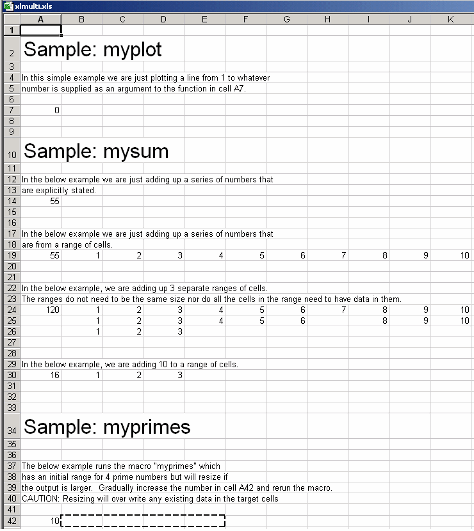## Create Macros from MATLAB Functions

### Create Add-Ins and Macros with Single and Multiple Outputs

The basic workflow for Microsoft® Excel® add-in and macro creation can be found in Create Excel Add-In from MATLAB and Integrate an Add-In and COM Component with Microsoft Excel.

### Work with Variable-Length Inputs and Outputs

This example shows you how to work with, and create macros from, functions having variable-length inputs and outputs

#### Overview

`myplot` takes a single integer input and plots a line from 1 to that number.

`mysum` takes an input of `varargin` of type `integer`, adds all the numbers, and returns the result.

`myprimes` takes a single integer input `n` and returns all the prime numbers less than or equal to `n`.

The Microsoft Excel file, `xlmulti.xls`, demonstrates these functions in several ways.

#### Create the Project

Use the following information as you work through this example using the instructions in Create Excel Add-In from MATLAB:

 Project Name `xlmulti` Class Name `xlmulticlass` File to compile (in the `xlmulti` folder of `myfiles\work`) `myplot.m` `myprimes.m` `mysum.m`

1. Start Microsoft Excel on your system.

2. Open the file `myfiles\work\xlmulti\xlmulti.xls`.

The example appears as shown:Note

If an Excel prompt says that this file contains macros, click Enable Macros to run this example.

#### Call myplot

This illustration calls the function `myplot` with a value of 4. To execute the function, make A7 (`=myplot(4)`) the active cell. Press F2 and then Enter.This procedure plots a line from 1 through 4 in a MATLAB® Figure window. This graphic can be manipulated similarly to the way one would manipulate a figure in MATLAB. Some functionality, such as the ability to change line style or color, is not available.

The calling cell contains 0 because the function does not return a value.

#### Call mysum Four Different Ways

This illustration calls the function `mysum` in four different ways:

• The first (cell A14) takes the values 1 through 10, adds them, and returns the result of 55 (`=mysum(1,2,3,4,5,6,7,8,9,10)`).

• The second (cell A19) takes a range object that is a range of cells with the values 1 through 10, adds them, and returns the result of 55 (`=mysum(B19:K19)`).

• The third (cell A24) takes several range objects, adds them, and returns the result of 120 (`=mysum(B24:K24,B25:L25,B26:D26)`). This illustration demonstrates that the ranges do not need to be the same size and that all the cells do not need a value.

• The fourth (cell A30) takes a combination of a range object and explicitly stated values, adds them, and returns the result of 16 (`=mysum(10,B30:D30)`).This illustration runs when the Excel file is opened. To reactivate the illustration, activate the appropriate cell. Then press F2 followed by Enter.

#### myprimes Macro

In this illustration, the macro `myprimes` calls the function `myprimes.m` with an initial value of 10 in cell A42. The function returns all the prime numbers less than 10 to cells B42 through E42.To execute the macro, from the main Excel window (not the Visual Basic® Editor), open the Macro dialog box, by pressing the Alt and F8 keys simultaneously, or by selecting Tools > Macro > Macros.

Select `myprimes` from the list and click .This function automatically resizes if the returned output is larger than the output range specified. Change the value in cell A42 to a number larger than 10. Then rerun the macro. The output returns all prime numbers less than the number you entered in cell A42.#### Inspect the Microsoft Visual Basic Code (Optional)

1. On the Microsoft Excel main window, select Tools > Macro > Visual Basic Editor.

2. On the Microsoft Visual Basic, in the Project - VBAProject window, double-click to expand `VBAProject (xlmulti.xls)`

3. Expand the `Modules` folder and double-click the `Module1` module. This opens the VB Code window with the code for this project.